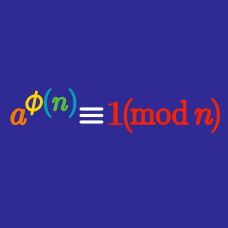Number Theory

# RSA

Solving which of the following problems would allow one to break RSA encryption?

Alice chooses a public key of $n=187$ and $e=3$. What is her private key?

Bob's public key is $426759435605268851$ and $e=3$. Alice uses ASCII encryption and sends the ciphertext $c=298049520771754739$. Which of the following was Alice's original message?

Mallory discovers many public keys, including the following:

• $n=70441807$, $e=3$
• $n=10645627$, $e=17$
• $n=63339281$, $e=65537$
• $n=24864431$, $e=257$
• $n=89221291$, $e=17$

What number can Mallory discover is the prime factor of one (or more) of these keys, without needing to factor any of them, making use of a vulnerability of RSA?

Knowing which of the following would allow an attacker to efficiently break the RSA encryption?

×